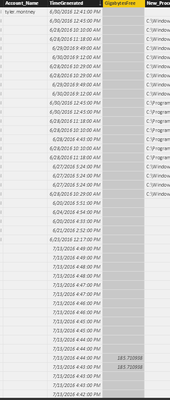cancel
Showing results for
Did you mean:Regular Visitor

## FIRSTNONBLANK returns blank

I want to return a columns first non blank value.

`LastLogon = FIRSTNONBLANK('TestReport'[Account_Name], 1) & " was the last to logon."`

Unfortunately, it returns nothing. I can verify the column I'm referring to has at least one value.1 ACCEPTED SOLUTIONMicrosoft

@tylermontney

The formula you provided should work if the rest value of column Account_Name is Blank(except value "tyler.montney"). Now the formula does not get the expected value, so I assume the rest value of the column Account_Name is not Blank, it is empty("").

In this case, the following formula should work:

```LastLogon =
CALCULATE (
FIRSTNONBLANK ( 'TestReport'[Account_Name], 1 ) & " was the last to logon.",
TestReport[Account_Name] <> ""
)```

3 REPLIES 3New Member
`FIRSTNONBLANK ( 'TestReport'[Account_Name], 1 ) +1`

use this query

I think this would be solved problemMicrosoft

@tylermontney

The formula you provided should work if the rest value of column Account_Name is Blank(except value "tyler.montney"). Now the formula does not get the expected value, so I assume the rest value of the column Account_Name is not Blank, it is empty("").

In this case, the following formula should work:

```LastLogon =
CALCULATE (
FIRSTNONBLANK ( 'TestReport'[Account_Name], 1 ) & " was the last to logon.",
TestReport[Account_Name] <> ""
)```Regular Visitor

How would you compare "blank" and "empty". As in null/nothing blank?

`PBDC-LT-16.PBDC.local,,12:45 june/30/2016,,C:\Windows\System32\cmd.exe,,`

I can see "empty" being a whitespace as a value. Above is an example of what blank should be (where a comma delimted value has absolutely nothing in it).